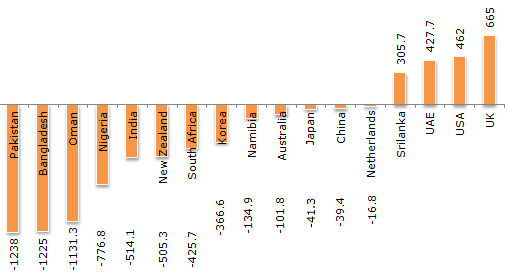# Data Interpretation - Bar Charts - Discussion

Discussion Forum : Bar Charts - Bar Chart 17 (Q.No. 5)
Directions to Solve

The total of the first three deficit countries (in Rs. crores) = 3594.3. The total of next five deficit countries (in Rs. crores) = 2588.5. The total of the last five deficit countries (in Rs. crores) = 334.2.

Cumulative Trade Deficit/Surplus of Countries for the Year 2006 - 2007 (All figures in Rs. Crores).5.
The net total deficit/surplus is equal to ?
4656.6 surplus
4656.6 deficit
3836.5 deficit
None of these
Explanation:
Adding all surpluses and subtracting all deficits will give an overall value of deficit of 4656.6.
Discussion:
5 comments Page 1 of 1.

Sanju said:   3 years ago
Total deficit is 6517.
Total surplus is 1860.4.

Payal said:   8 years ago
Total deficit is 6516.9.

And Total surplus is 1860.4.

Sandy said:   8 years ago
Total deficit = 6516.

Total surplus = 1800.

Difference = 4716 deficit.

Aqwer said:   9 years ago
Sir my total surplus is coming out to be 1860.4 and total deficit is coming out to be -6889, so the overall deficit/surplus is -5028.6.

Kindly explain how the answer to this question is option B.